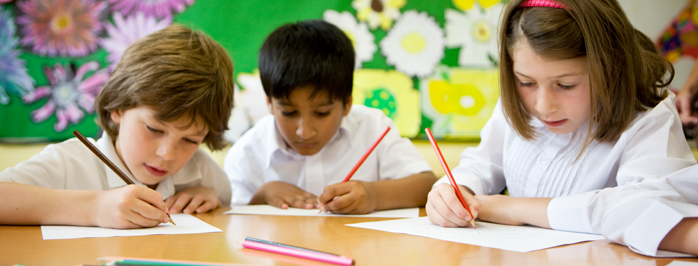## Math Worksheets for Kindergarten, 1st, 2nd, 3rd, 4th grade+

Rocket Math worksheets are a great way to teach math facts to children of all ages – starting as early as kindergarten when students begin learning how to count and read and write numbers. The many learning tracks available in the Worksheet Program are designed for daily practice to build a solid foundation of basic math skills.

The learning tracks of the Universal Level Worksheet Program follow a simple structure and routine to help students progress at an appropriate rate throughout their different grade levels. Throughout the sequence, students learn the underlying skills necessary to be successful in any math curriculum throughout elementary and middle school. While Rocket Math Worksheets are not a complete math curriculum, they develop all the skills that are assumed, but seldom taught well in elementary math curricula.

If you have students behind for their grade level, our worksheet program makes it easy for you to revisit previous lessons that will reinforce the concepts necessary to move forward. Likewise, plenty of learning tracks within the program keep advanced students engaged.

## Kindergarten Math Worksheets

### Beginning Numerals (Counting) Learning Track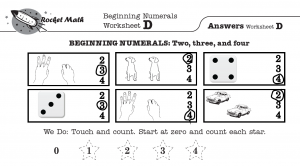This is a beginning program for kindergarten students.  It teaches them to count objects aloud and then match the word with the numeral. Please note: Unlike most math worksheets learning tracks, this program is teacher-directed rather than peer teaching.

Each worksheet begins with a demonstration of counting objects and circling the numeral that matches. On Worksheet A, students only need to learn numbers two and three. First, the teacher demonstrates (best with a document camera so all students can see) how to count the objects and then points out that the answer has a circle around it.  In the “We do” portion of the worksheet, the teacher counts the stars first as a demo and then with the students. For Worksheet A, just count 3 stars. By Worksheet S, the teacher and the students are counting 12 stars together. In the “You do” portion, the students independently complete tasks like the ones demonstrated by the teacher at the top of the worksheet.  By the end of this learning track, kindergarteners can consistently count objects, lines, dots, and fingers up to 12 and identify the corresponding numeral.

### Conceptual Addition (Counting On) Learning Track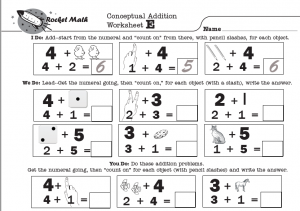Conceptual Addition is a teacher-led exercise, rather than peer teaching. Each worksheet begins with an “I Do” portion that is a teacher-modeled demonstration. “I Do” is followed by a “We Do” portion where the teacher leads the students in doing the problems together. The last portion is called “You Do,” which students should be able to complete independently following teacher instruction and modeling.

For the first four worksheets, the objective is to count all the objects in two groups to find the sum. When modeling, teachers should slash the objects with a pencil while counting. Students should do the same. The answer keys show the objects slashed. Starting in Set E, counting exercises no longer begin at one nor progress one by one. Students learn the concept of “counting on.” “Counting on” means to start with a number such as “4” and “count on” from there (five, six, and so on). Students also learn the concept of adding two to four by starting with four and “counting on” two more.

### Rocket Writing for Numerals Learning Track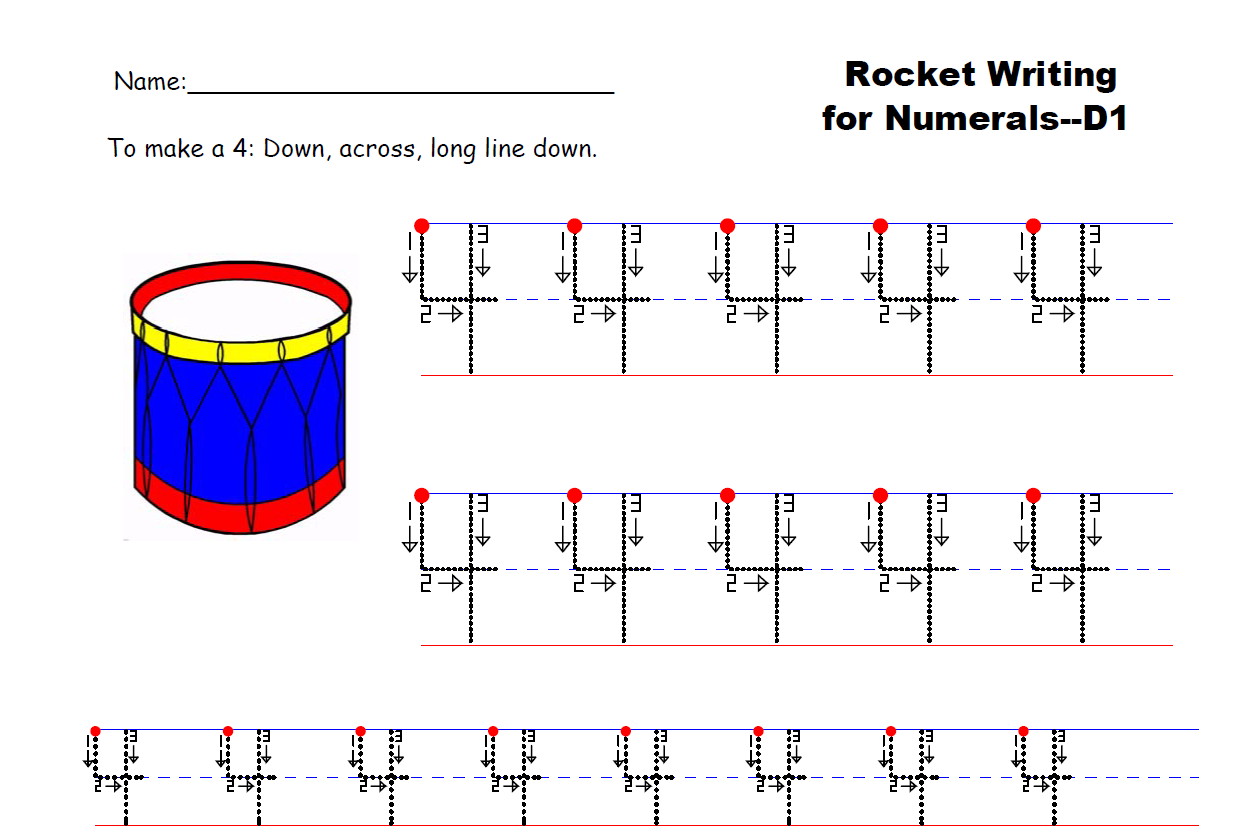One of the most important math-related skills for kindergarteners is to learn how to write numerals properly. It is important for children to learn the most efficient way to write numerals. This skill is the first building block to learning math.

Rocket Writing for Numerals is a 72-page learning track for students to learn how to write the numerals efficiently. This program begins with how to write numerals and continues until students can write 40 digits in a minute. It is part of the Rocket Math Universal Worksheet Program and is designed to be practiced on a daily basis.

If students in first grade cannot write numerals legibly and efficiently, they should begin the year with Rocket Writing for Numerals. If students have not learned the concept of what it means to add in kindergarten with the Conceptual Addition learning track, that is recommended. Once they understand the concept of addition, first graders are ready to begin memorizing addition facts.

### Addition 0 through 9s Learning Track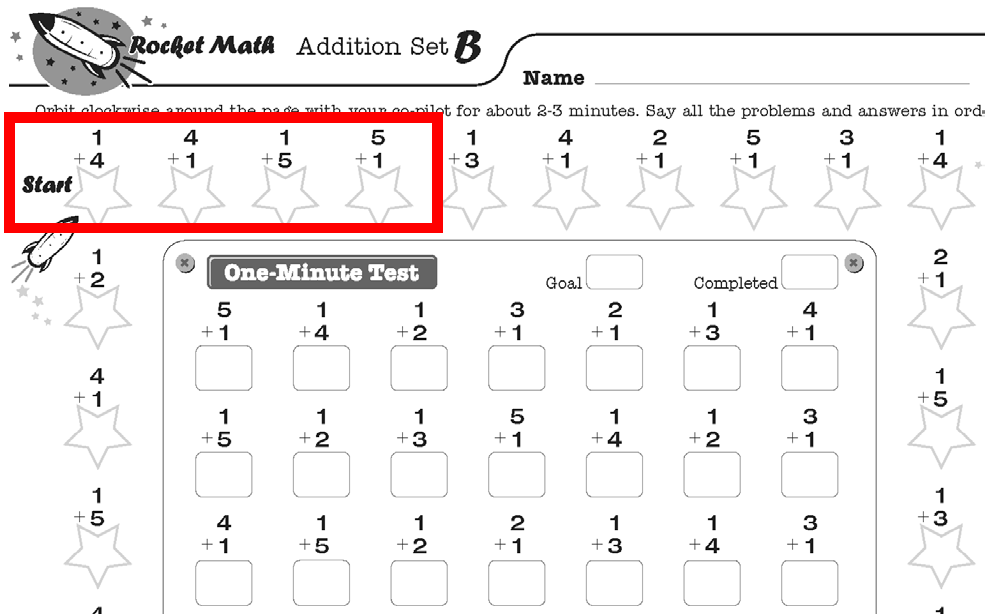The Rocket Math Worksheet Program includes Addition 0 through 9s. Students work through 26 levels (A to Z) learning two facts and their reverses on each level. For this learning track, like most of the learning tracks, students practice orally for 2 minutes with a partner who corrects any hesitations or errors.  The students then switch roles and practice again, followed by a 1-minute daily test to see if they are ready to move on to the next worksheet. For peer teaching like this to be effective, the teacher will need to teach their students how to practice and how to correct errors. Here’s a blog on how to best manage peer teaching.

### Add-Subtract Fact Families to 10 Learning Track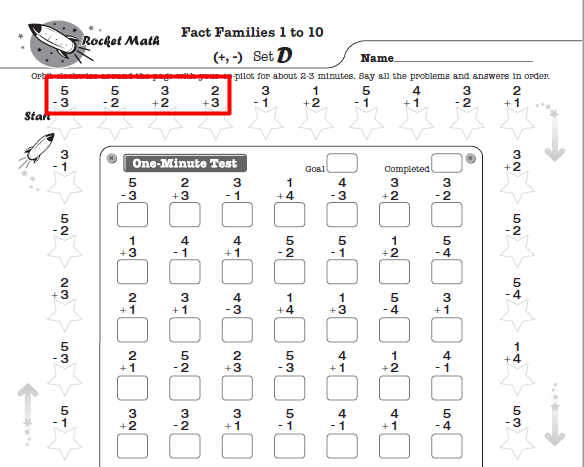Alternatively, once students have learned the concepts for both addition and subtraction, they can begin to learn fact families. Our Add-Subtract Fact Families to 10 worksheet program begins teaching fact families. For example, Set D of this worksheet (pictured) teaches the four related math facts of a fact family such as 3+2, 2+3, 5-2, and 5-3.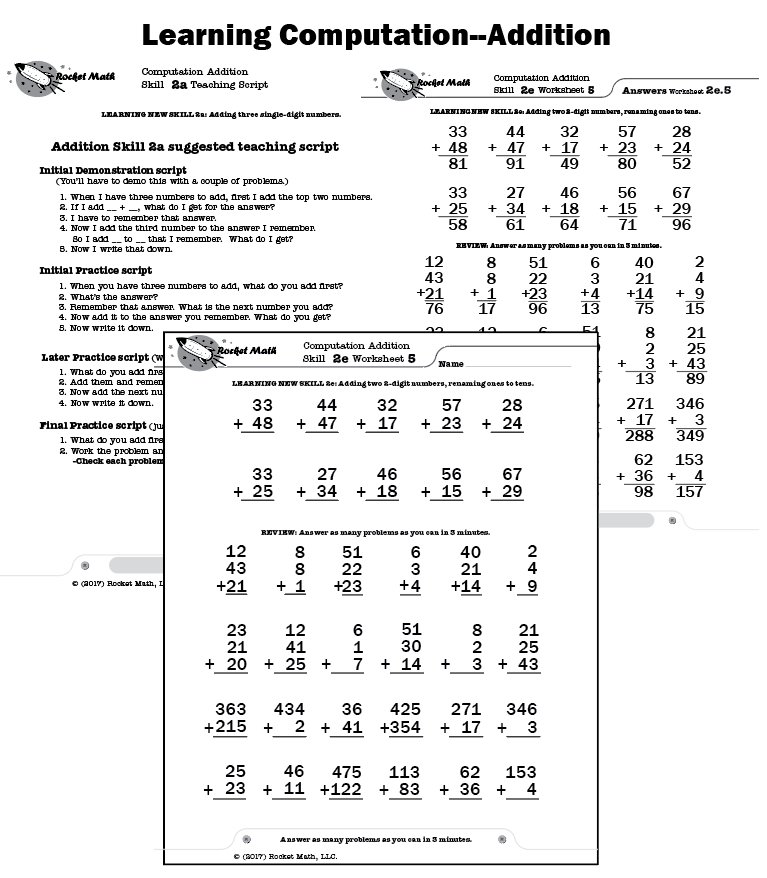Once students master addition math facts, they need to learn how to do multi-digit addition problems, such as adding 35 + 48. Teachers can start their students on the Addition-Learning Computation learning track. This learning track extends computational skills in addition up to the 4th grade. It is teacher-directed and includes suggested scripts for tutoring or small group instruction. There is an assessment to determine where to begin, although, for first-grade students, it is at the beginning.

### Add to 20 Learning Track

Common Core suggests that students be fluent with addition math facts up to 20, such as 13+6=19. Rocket Math’s Universal subscription includes an Add to 20 learning track.

Upon starting second grade, students should have mastered all of the addition math facts. If they have not mastered those facts, teachers should have students start with the first-grade worksheets until they are ready to move forward.

### Subtraction 0 through 9s Learning Track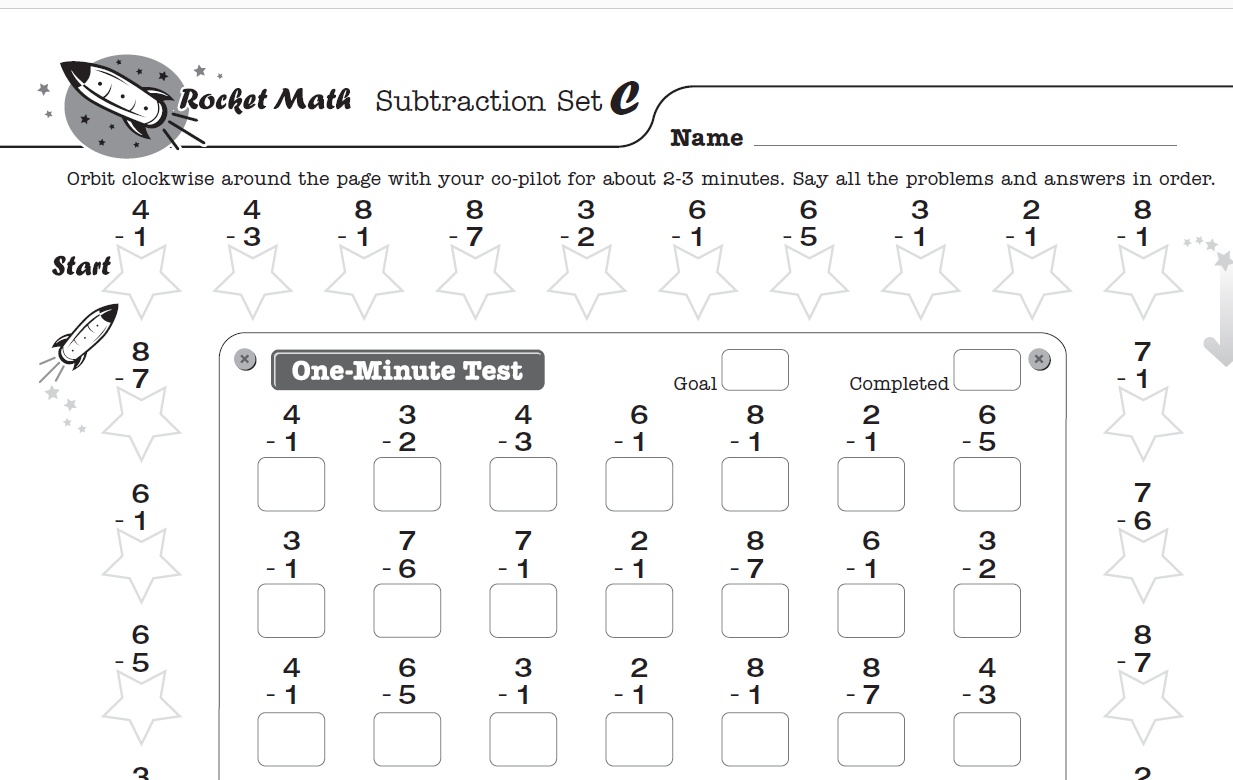Once students master the first-grade level learning tracks, second-grade students go on to memorize subtraction facts in the Subtraction 0 through 9s learning track. For this learning track, like most of the learning tracks, students practice orally for 2 minutes with a partner who corrects any hesitations or errors.  The students then switch roles and practice again, followed by a 1-minute daily test to see if they are ready to move on to the next worksheet. For peer teaching like this to be effective, the teacher will need to teach their students how to practice and how to correct errors. Here’s a blog on how to best manage peer teaching.

### Subtraction-Learning Computation Learning Track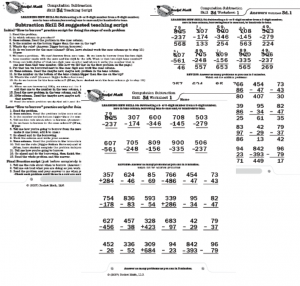Typically, second graders should be proficient in subtraction and addition by the end of the year. Once students master subtraction math facts, students need to learn how to do multi-digit subtraction problems such as 62 – 48. Teachers can start their students on the Subtraction-Learning Computation learning track. The program helps students learn re-grouping (a.k.a. “borrowing”). This learning track extends computational skills in subtraction up to the 4th grade. It is teacher-directed and includes suggested scripts for tutoring or small group instruction. There is an assessment to determine where to begin, although, for second-grade students, it is at the beginning.

### Add-Subtract Fact Families 11 to 18 Learning Track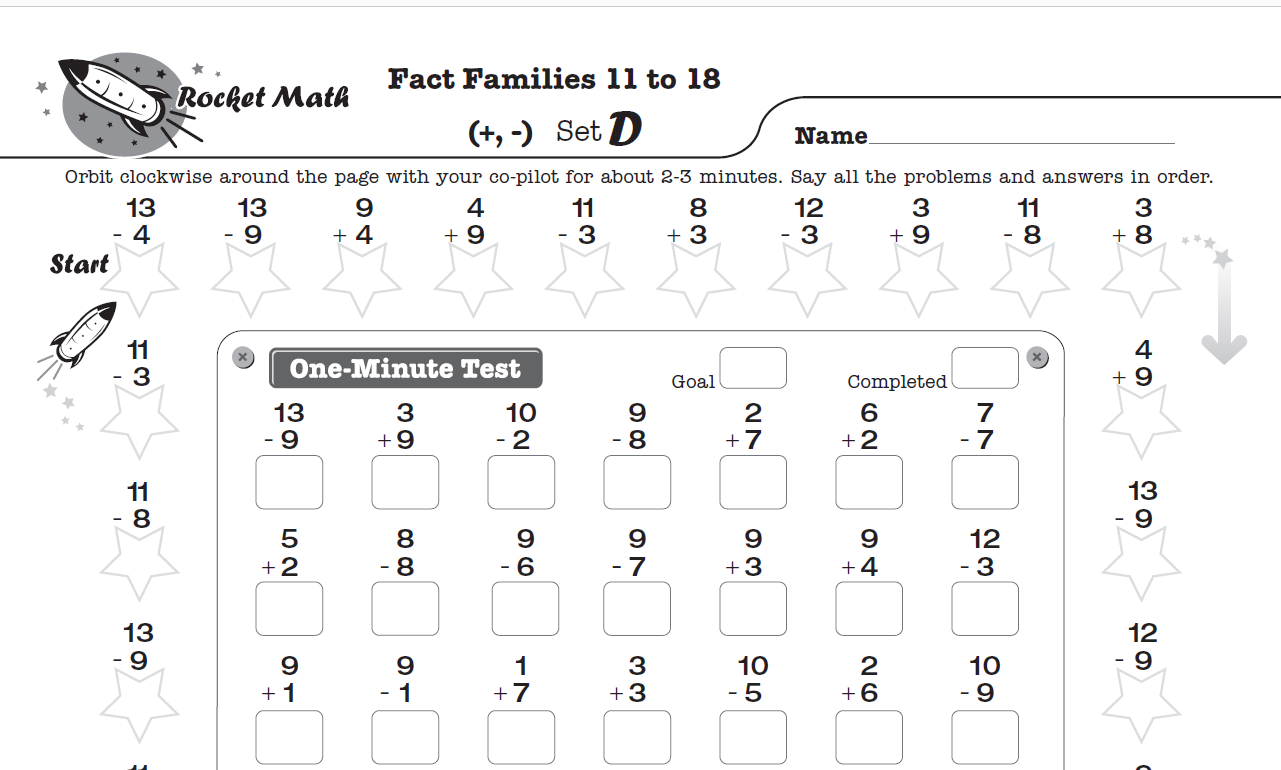If students have been using the Fact Families Program in first grade, it is best to continue using that sequence. Second graders should be ready for Add-Subtract Fact Families 11 to 18. The last ten levels of this program review all the fact families, allowing students to master addition and subtraction by the time they reach Level Z.

### Subtract from 20 Learning Track

Common Core suggests that students be fluent with subtraction facts up to 20, such as 19-6=13. Rocket Math has made available a program, Subtract from 20, so students can practice these facts.

The priority for third grade is to learn the concept of multiplication and then memorize those facts. Textbooks typically begin teaching the concept of multiplication from very early in 3rd grade.

###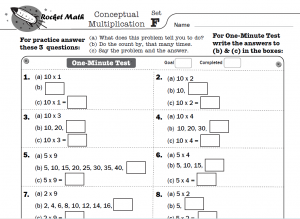Conceptual Multiplication is a learning track to teach the concept of multiplication. It is based on skip counting or count-bys. However, in this learning track, students can read all the count-by series except the last number, making it unnecessary for students to have memorized the skip counting sequences to do this learning track.

For this learning track, like most of the learning tracks, students practice orally for 2 minutes with a partner who corrects any hesitations or errors.  The students then switch roles and practice again, followed by a 1-minute daily test to see if they are ready to move on to the next worksheet. For peer teaching like this to be effective, the teacher will need to teach their students how to practice and how to correct errors. Here’s a blog on how to best manage peer teaching.

Conceptual Multiplication is essential for students who have not been introduced to multiplication in their regular math curriculum but are otherwise ready to start memorizing multiplication facts. Students do NOT need this learning track if they can determine the answer to any multiplication fact when you ask them, for example, “What’s seven times eight?” If they can figure out the answer to that question, they already understand the concept of multiplication and can go on to memorizing.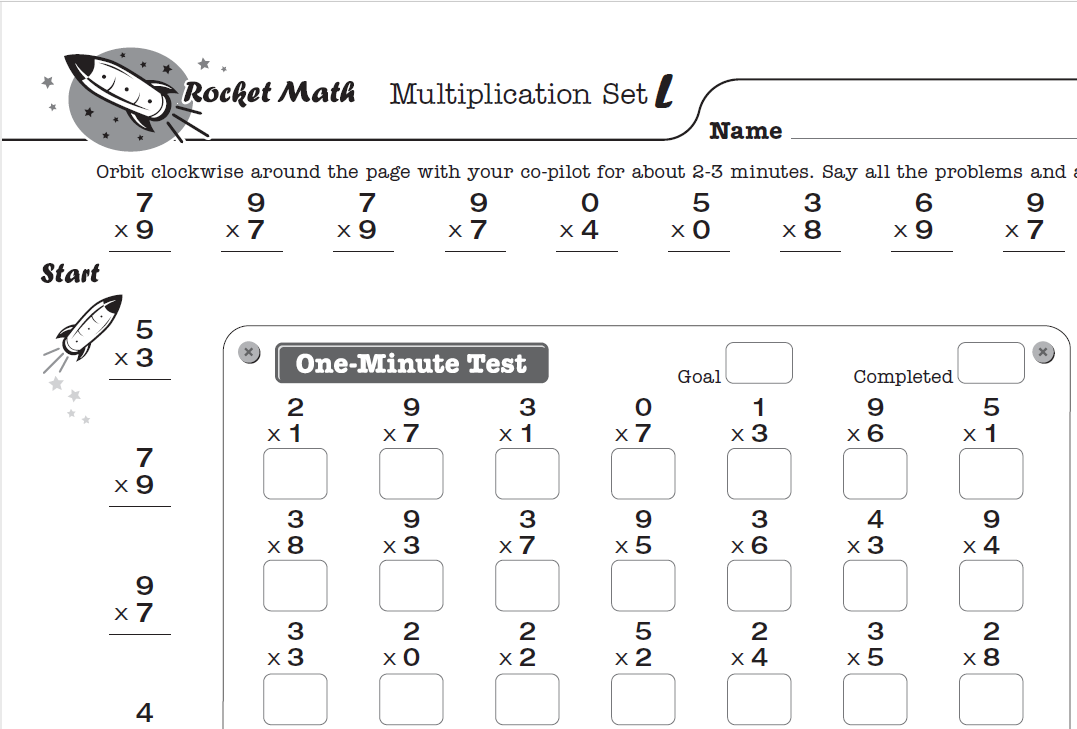### Multiplication (0 through 9s) Learning Track

The Rocket Math Multiplication (0 through 9s) Learning Track builds strong multiplication fact fluency and recall. This learning track prevents the problem of students having to look up facts in times tables repeatedly.

Achieving mastery of multiplication facts is the only way for students to keep up in math throughout elementary and middle school. Even if students come to you without having a solid foundation in addition and subtraction facts, your top priority must be to help students develop fluency in multiplication facts before they leave you.

### Multiply-Divide Fact Families to 20 Learning Track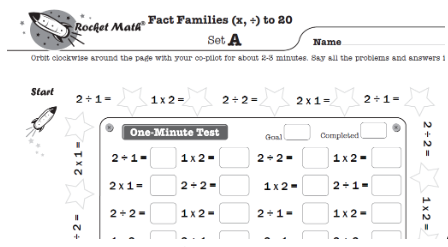Alternatively, once students have learned the concepts for both multiplication and division, they can begin to learn fact families. Our Multiply-Divide Fact Families to 20 worksheet program begins teaching multiplication and division fact families. For example, Set A of this worksheet (pictured) teaches the four related math facts of a family:  2÷1=2, 1×2=2, 2÷2=1, and 2×1=2.

###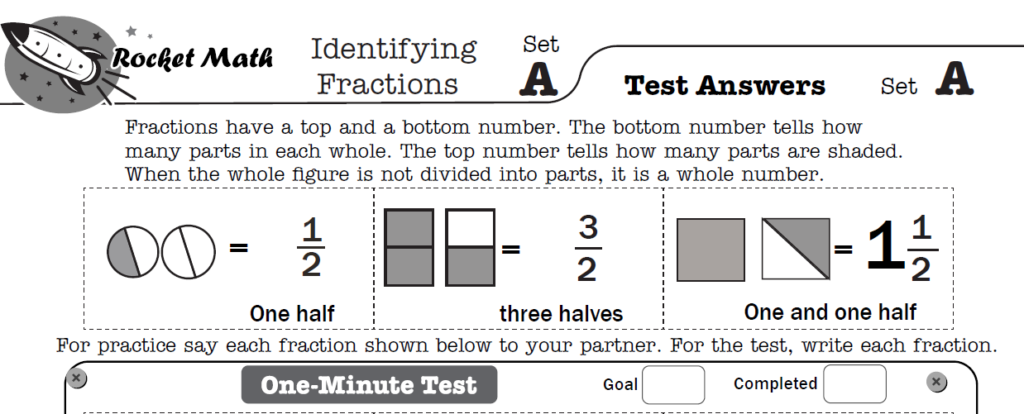For quick-learning students, who get through the basic learning track before the end of the year, an excellent supplemental Rocket Math Worksheet Program is the Identifying Fractions learning track. This is appropriate any time from third grade up through fifth.  This learning track teaches students how to identify proper fractions, improper fractions and mixed numbers as you can see in the example above from Set A. It teaches fractions with denominators up to twelfths.  This learning track prevents  the most common misunderstandings about fractions by teaching with carefully selected examples that demonstrate the correct understanding of fractions.

### Multiplication 10s, 11s,12s Learning Track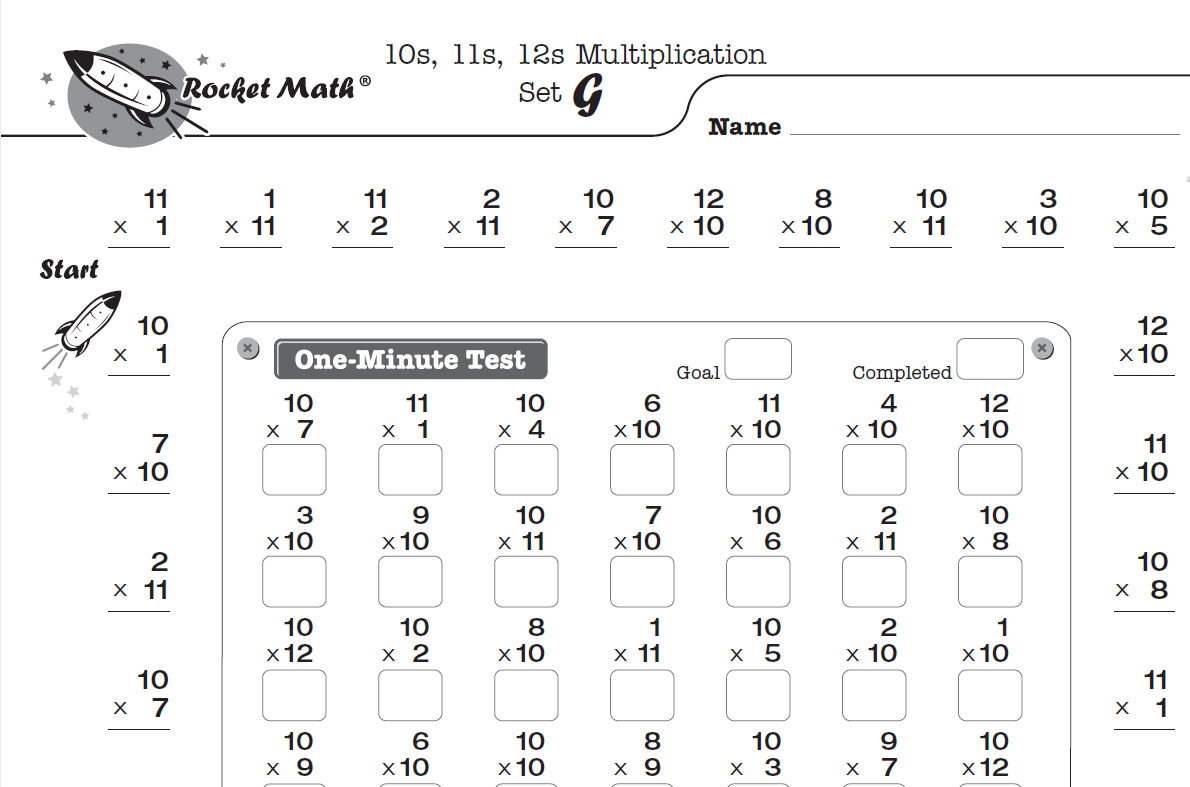Multiplication 10s, 11s, 12s. Building upon the facts from Multiplication 1s to 9s, students progress quickly through this next set of facts. And, of course, students consider it a badge of honor to be members of the “tens, elevens, and twelves” club!

### Multiplication-Learning Computation Learning Track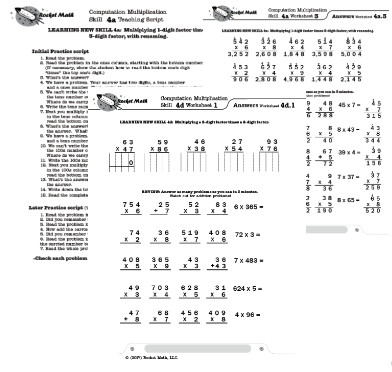Once students master multiplication math facts, they need to learn how to do multi-digit multiplication problems such as multiplying 6 x 37. Teachers can start their students on the Multiplication-Learning Computation learning track. This learning track extends computational skills in multiplication up to the 5th grade. It is teacher-directed and includes suggested scripts for tutoring or small group instruction. There is an assessment to determine where to begin, although, for third-grade students, it is at the beginning.

Upon starting fourth grade, students should have mastered all of the multiplication math facts. If they have not mastered those facts, teachers should have students start with the multiplication worksheets until they are ready to move forward into division. While division facts derive from multiplication, it is valuable for students to learn the division facts explicitly.

###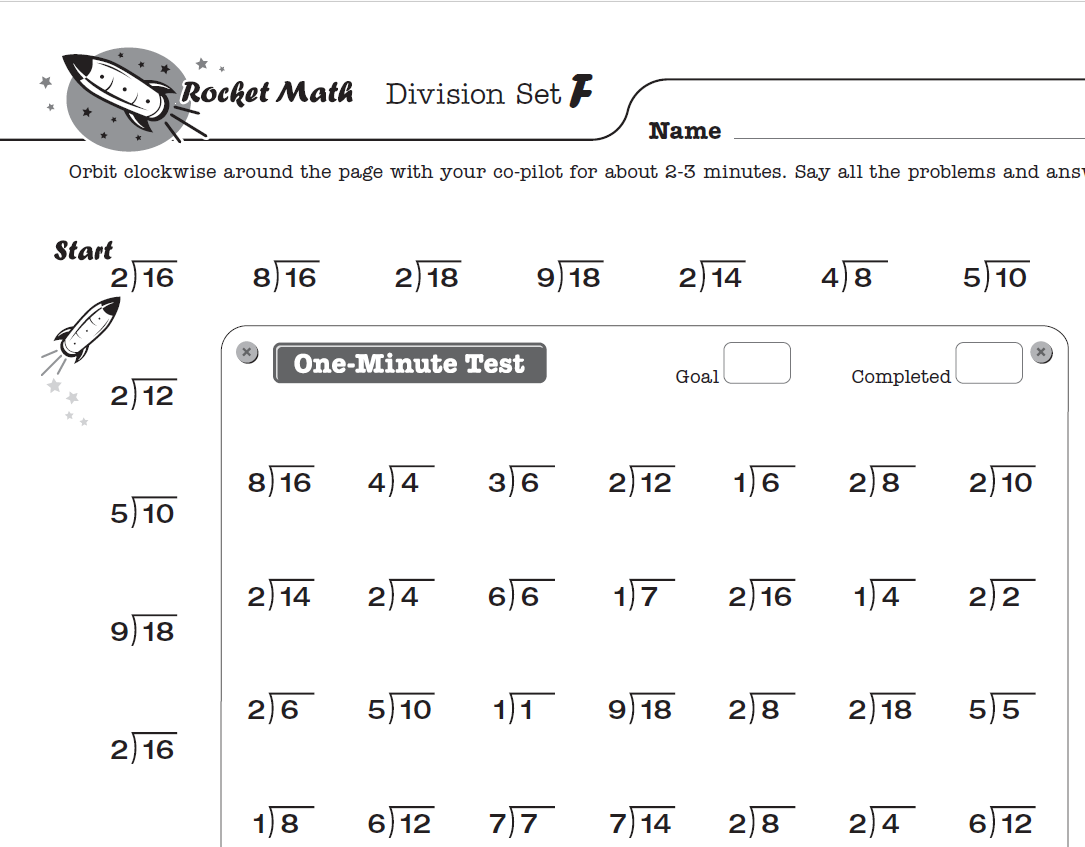Long division comes much easier for students who have learned division facts. Fourth graders should be using the Division 0 through 9s worksheet to learn division facts. By the end of fourth grade, students should have learned all four basic operations of math facts.

### Multiply-Divide Fact Families from 21 Learning Track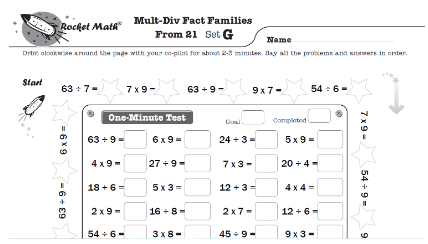If students have been using the Multiplication-Division Fact Families Program in third grade, it is best to continue using that sequence. Fourth graders should be ready for Multiply-Divide Subtract Fact Families from 21. The last several levels of this program review all the fact families, allowing students to master all multiplication and division facts by the time they reach Level Z.

### Division-Learning Computation Learning Track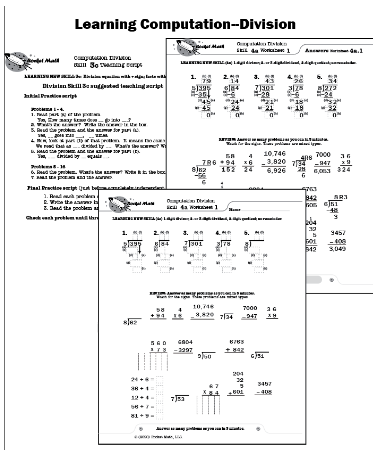Once students master division math facts, they need to learn how to do multi-digit division problems such as dividing 5 into 395.  Teachers can start their students on the Division-Learning Computation learning track.   This learning track extends computational skills in multiplication up to the 4th grade. It is teacher-directed and includes suggested scripts for tutoring or small group instruction. There is an assessment to determine where to begin.

### Division 10s, 11s, 12s Learning Track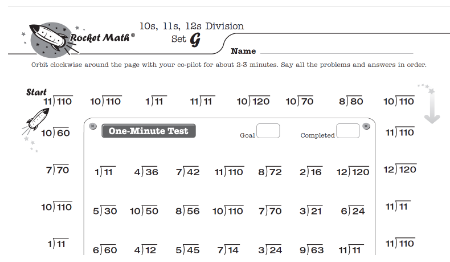There is a supplemental program for students who get through Level Z in basic division: Division 10s, 11s, 12s.  It builds upon and reviews facts from Division 0 to 9s while keeping learning fun for students who learn faster.

## Math Worksheets for 5th grade and up

Students who have been learning math facts since kindergarten have built a great foundation by the time they reach fifth grade. Unfortunately, this is not the case for many students, which makes it difficult for teachers to create lessons suitable for everyone’s level.

Rocket Math provides an easy solution for these teachers. Utilize the worksheet program for ten minutes daily to teach the four basic operations. While these students are catching up, the remaining students can also do Rocket Math!

We offer five learning tracks that teach more advanced pre-algebra math skills that follow the same structure and routine. Each of these five learning tracks includes video lessons that teach students how to use the program and how to do the skill. If students have not done the learning track Identifying Fractions [presented above in grade 3 Worksheet options], we recommend students do that first, before beginning the Pre-Algebra Learning Tracks.

###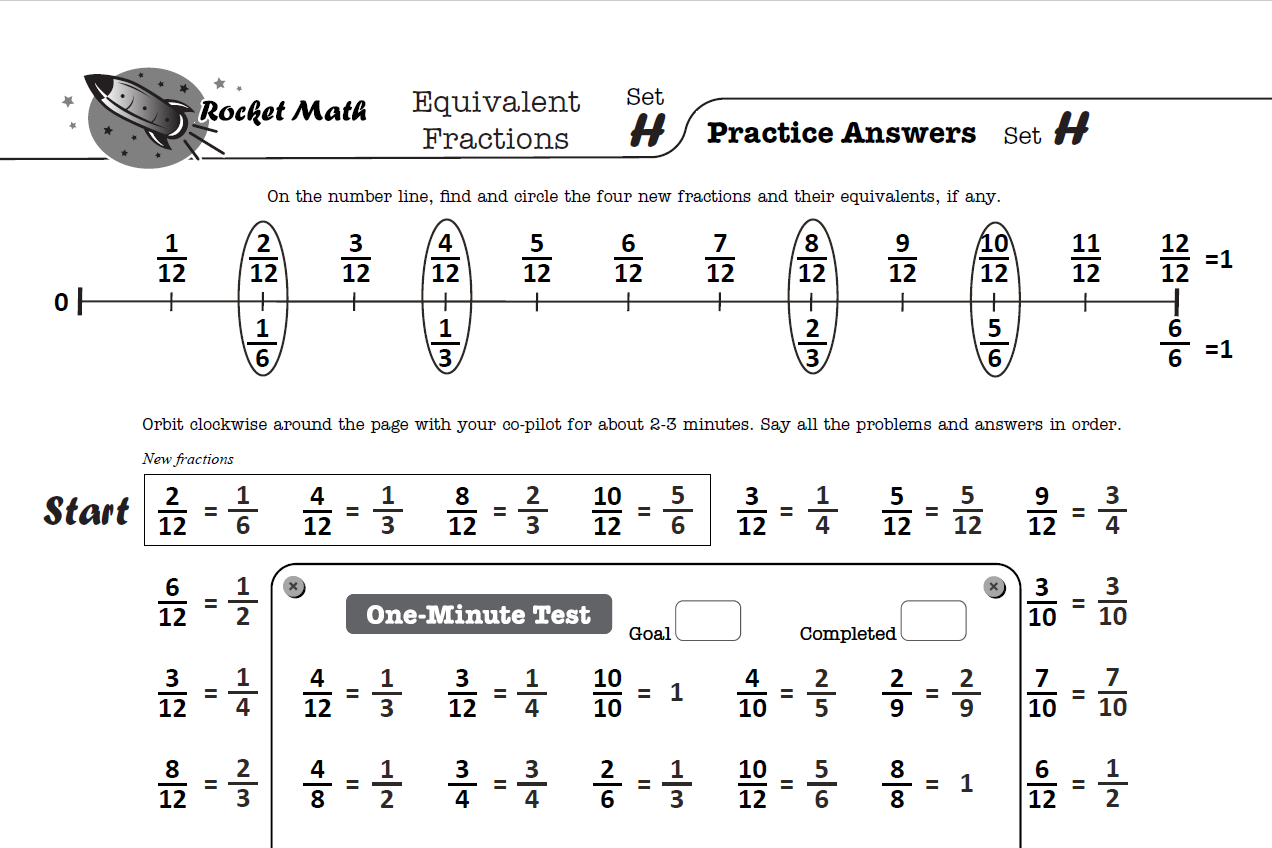2) Factors

3) Learning to Add Integers (positive and negative numbers)

4) Learning to Subtract Integers (positive and negative numbers)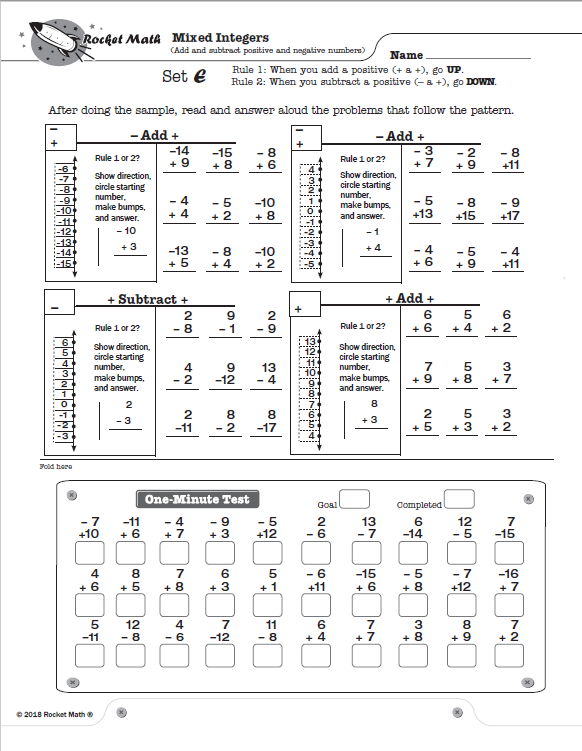5) Mixed Integers (adding and subtracting positive and negative numbers)

Rocket Math’s goal is to make learning fun for everyone–students and teachers. By following the Worksheet Program, even students who have struggled in the past can begin gaining confidence in their math skills.

Because the Rocket Math Worksheet program follows a sequence and routine, it is easy for students to continue working together even when studying different programs or levels.

With the right underlying skills, students can enjoy learning math, and teachers can relax knowing that a strong foundation is forming. Our FAQs page for Teacher Instructions and How to Implement the Worksheet Program can help answer any questions you might have.

Ready to get started? Subscribe to Rocket Math’s Worksheet program today. It’s available for individual students and entire school districts.

## 4 thoughts on “Math Worksheets for Kindergarten, 1st, 2nd, 3rd, 4th grade+”

1.Tara Reed says:

Hello my name is Tara Reed and i have a kindergarten and a third grader. How can i print out pages for Rocket Math facts

2.Arayah Durbin says:

This is awsome!

•Admindon says:

Glad you like it. I need to update this blog as we have three more Learning Tracks, two in Kindergarten level and one for second or third grade.